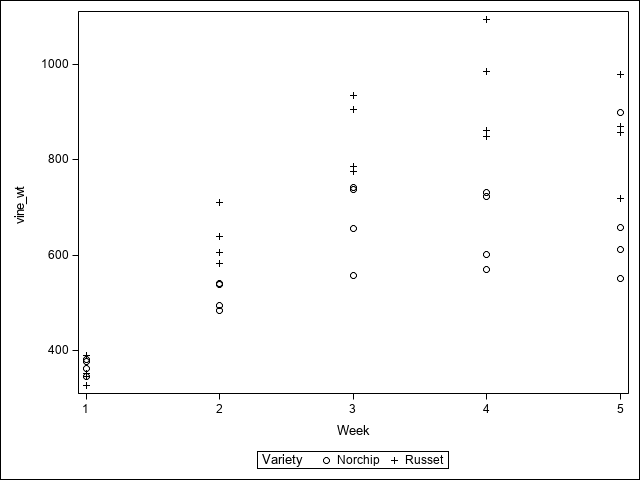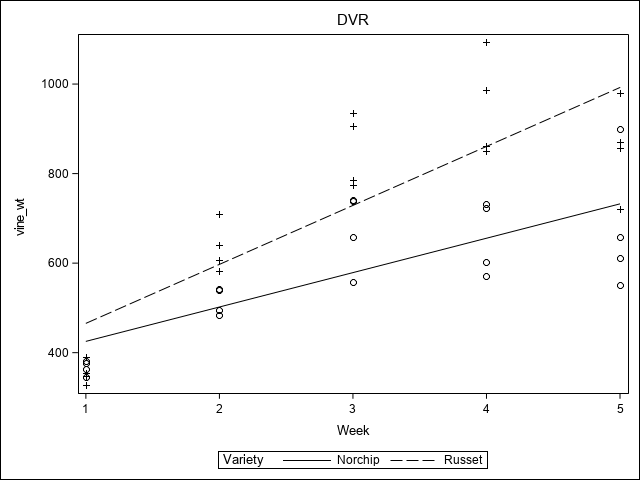# 1 Introduction

This tutorial will cover Analysis of Covariance and Dummy Variable Regression (DVR) using SAS. Analysis of Covariance (ANCOVA) and DVR are linear models that combine the characteristics of ANOVA and Regression. While ANOVA uses all discrete, categorical factors and regression uses all continuous variables, ANCOVA and DVR models are a combination of both discrete factors and continuous regressors.

Both ANCOVA and DVR have the same or very similar structure mathematically. They differ, however, in their interpretation. Analysis of Covariance aims at adjusting the estimation and comparisons of discrete factors through a linear relationship with an additional continuous variable or covariate. For example, an analysis might seek to compare average daily gain (ADG) in calves for various types of feed rations (factors). ADG, however, can also be related to the initial body weight of the calves. The analysis, then, could fit the discrete rations as factors, while simultaneously adding initial body weight to the model as a continuous covariate. The resulting mean estimates and comparisons from the model would then be adjusted for initial body weight. Doing the analysis in this manner is a post-hoc method of accounting for a potential confounding effect. To be effective, ANCOVA assumes the covariate is linearly related to the response variable. In addition, it is best to have unique covariate values for every experimental unit. Lastly, multiple covariates are possible, however, care should be taken to avoid using too many and considering whether the covariates may interact of be related to one another.

Dummy Variable Regression, on the other hand, focuses on the linear relationship with the covariate. That is, we want to compare regression relationships across the discrete levels of the factors. An example might be comparing the biomass response of a crop to nitrogen rates (continuous covariate) across several varieties (discrete factor). As with ANCOVA, there is an assumption that the relationship with the covariate is linear. We also want to take caution in comparing too many levels of a factors, e.g. comparing 3-4 varieties as opposed to comparing 30 varieties. for details on how Dummy Variable regression model work see the DVR section of the Regression tutorial.

For more detailed information on mixed models, ANOVA, Regression, DVR, and ANCOVA in SAS, the readers are referred to Claassen et al. (2018).

# 2 Data used in examples

This tutorial will use one set of data to illustrate both ANCOVA and DVR. The data describe a potato variety trial (2 varieties) and measure above ground vine weight over 5 weeks in a blocked experimental design. A CSV file for this data can be found here and example code to read in and plot the data is shown below. For more information on reading data into SAS, please see the tutorial on the SAS Data Step.


proc import out= work.vine
datafile= ".\data\vine_wt.csv"
dbms=csv replace;
run;

proc sgplot data=vine;
scatter x=week y=vine_wt/group=variety;
run;# 3 Analysis of Covariance

Both an ANOVA model and an ANCOVA model are demonstrated below. The covariance model is set up in the same manner as an ANOVA model, with the addition of the covariate (Week, in this case) as a fixed effect. The important aspect here is that Week is not in the ANCOVA CLASS statement. This causes Week to enter the model as the numeric values: 1, 2, 3, 4, and 5.


proc mixed data=vine;
class block variety;
model vine_wt = variety;
random block ;
lsmeans variety;
title1 'ANOVA';
run;

proc mixed data=vine;
class block variety;
model vine_wt = variety week;
random block ;
lsmeans variety;
title1 'ANCOVA';
run;
 ANOVA

Model Information
Data Set WORK.VINE
Dependent Variable vine_wt
Covariance Structure Variance Components
Estimation Method REML
Residual Variance Method Profile
Fixed Effects SE Method Model-Based
Degrees of Freedom Method Containment

Class Level Information
Class Levels Values
Block 4 1 2 3 4
Variety 2 Norchip Russet

Dimensions
Covariance Parameters 2
Columns in X 3
Columns in Z 4
Subjects 1
Max Obs per Subject 40

Number of Observations
Number of Observations Used 40
Number of Observations Not Used 0

Iteration History
Iteration Evaluations -2 Res Log Like Criterion
0 1 514.21617867
1 1 514.21617867 0.00000000

 Convergence criteria met.

Covariance Parameter Estimates
Cov Parm Estimate
Block 0
Residual 37664

Fit Statistics
-2 Res Log Likelihood 514.2
AIC (Smaller is Better) 516.2
AICC (Smaller is Better) 516.3
BIC (Smaller is Better) 515.6

Type 3 Tests of Fixed Effects
Effect Num DF Den DF F Value Pr > F
Variety 1 35 5.98 0.0196

Least Squares Means
Effect Variety Estimate Standard
Error
DF t Value Pr > |t|
Variety Norchip 578.93 43.3958 35 13.34 <.0001
Variety Russet 729.01 43.3958 35 16.80 <.0001

 ANCOVA

Model Information
Data Set WORK.VINE
Dependent Variable vine_wt
Covariance Structure Variance Components
Estimation Method REML
Residual Variance Method Profile
Fixed Effects SE Method Model-Based
Degrees of Freedom Method Containment

Class Level Information
Class Levels Values
Block 4 1 2 3 4
Variety 2 Norchip Russet

Dimensions
Covariance Parameters 2
Columns in X 4
Columns in Z 4
Subjects 1
Max Obs per Subject 40

Number of Observations
Number of Observations Used 40
Number of Observations Not Used 0

Iteration History
Iteration Evaluations -2 Res Log Like Criterion
0 1 471.59110040
1 1 471.59110040 0.00000000

 Convergence criteria met.

Covariance Parameter Estimates
Cov Parm Estimate
Block 0
Residual 15176

Fit Statistics
-2 Res Log Likelihood 471.6
AIC (Smaller is Better) 473.6
AICC (Smaller is Better) 473.7
BIC (Smaller is Better) 473.0

Type 3 Tests of Fixed Effects
Effect Num DF Den DF F Value Pr > F
Variety 1 34 14.84 0.0005
Week 1 34 57.31 <.0001

Least Squares Means
Effect Variety Estimate Standard
Error
DF t Value Pr > |t|
Variety Norchip 578.93 27.5462 34 21.02 <.0001
Variety Russet 729.01 27.5462 34 26.46 <.0001

In the ANCOVA analysis, the Type 3 test for Week has a low p-value and, hence, appears to be relevant to the analysis. Note that Week has 1 degree of freedom. This should always be the case for covariates. If you see more degrees of freedom, then likely you have used the covariate in the CLASS statement. The estimated ANCOVA LSMeans are identical to those from ANOVA, however, the standard errors for the ANCOVA means are substantially smaller than the ANOVA version. The Analysis of Covariance is providing higher precision estimates because those means are now adjusted for Week, as are any associated tests. The use of the covariate has cost 1 DF relative to the ANOVA (35 df to 34), however, the low p-value for the covariate and the increased precision of the LSMeans would justify this cost.

# 4 Dummy Variable Regression

In the following example, a similar model is used with the addition of an interaction term, Variety*Week. There are two options also added to the MODEL statement for solution and noint. In this model, the main effect of Variety codes for the regression intercept coefficients of each variety. The interaction term codes for the respective slope coefficients. This is called the Full Model because it allows for all intercepts and all slopes to be independently estimated. Because Proc Mixed attempts to insert an overall intercept term by default, and we have already specified a term for intercepts, the noint option is used to suppress the overall default value. Also by default, SAS does not print the coefficients for factors in the Proc Mixed output. Because of this, the solution option is used to force them to be printed. These will be the intercept and slope estimates. The last option, outp=pred, tells SAS to save the predicted values in a new data set Pred. This data set will have all the original data, in addition to the predicted values and their standard errors. That data is then used after Proc mixed to plot and visualize the predicted lines for each Variety. The comparison of the two regression lines for each variety occurs in the Contrast statements. The lines can be compared in several ways. We can ask if the intercepts are equivalent (contrast #1). We can also ask if the slopes or rate of change over Weeks is the same for each variety (contrast #2). Or, lastly, we can ask if both intercepts and slopes are equivalent (contrast #3, coincidence of lines).


proc mixed data=vine;
class block variety;
model vine_wt = variety variety*week/solution noint outp=pred;
random block ;
contrast 'Intercepts' Variety 1 -1;
contrast 'Slopes' Variety*Week 1 -1;
contrast 'Lines' Variety 1 -1, Variety*Week 1 -1;
title1 'DVR';
run;

proc sgplot data=pred;
series x=week y=pred/group=variety;
scatter x=week y=vine_wt/group=variety;

run;
 DVR

Model Information
Data Set WORK.VINE
Dependent Variable vine_wt
Covariance Structure Variance Components
Estimation Method REML
Residual Variance Method Profile
Fixed Effects SE Method Model-Based
Degrees of Freedom Method Containment

Class Level Information
Class Levels Values
Block 4 1 2 3 4
Variety 2 Norchip Russet

Dimensions
Covariance Parameters 2
Columns in X 4
Columns in Z 4
Subjects 1
Max Obs per Subject 40

Number of Observations
Number of Observations Used 40
Number of Observations Not Used 0

Iteration History
Iteration Evaluations -2 Res Log Like Criterion
0 1 459.01382401
1 1 459.01382401 0.00000000

 Convergence criteria met.

Covariance Parameter Estimates
Cov Parm Estimate
Block 0
Residual 13921

Fit Statistics
-2 Res Log Likelihood 459.0
AIC (Smaller is Better) 461.0
AICC (Smaller is Better) 461.1
BIC (Smaller is Better) 460.4

Solution for Fixed Effects
Effect Variety Estimate Standard
Error
DF t Value Pr > |t|
Variety Norchip 348.53 61.8726 33 5.63 <.0001
Variety Russet 333.80 61.8726 33 5.39 <.0001
Week*Variety Norchip 76.7985 18.6553 33 4.12 0.0002
Week*Variety Russet 131.73 18.6553 33 7.06 <.0001

Type 3 Tests of Fixed Effects
Effect Num DF Den DF F Value Pr > F
Variety 2 33 30.42 <.0001
Week*Variety 2 33 33.41 <.0001

Contrasts
Label Num DF Den DF F Value Pr > F
Intercepts 1 33 0.03 0.8674
Slopes 1 33 4.34 0.0451
Lines 2 33 10.26 0.0003In the output, we can see in the Solution for Fixed Effects table that the intercepts are 348 and 333 for Norchip and Russet, respectively, while the slopes were 76 and 131, respectively. The contrast results give us more information. There is no detectable difference in the intercept terms and, even though the slopes differ by almost 2x, there is weak evidence that they are different. The overall test of lines does show a strong difference. This is likely becuase the lines contrast has 2 DF and looks at both intercepts and slopes simultaneously, while the individual tests are less powerful with 1 DF and carried out independently. Overall there is some evidence that the lines differ and that difference is due to a larger slope value for Russet. The plot that follows demonstrates this with lines overlaying the data points.